## Tensor张量基础与常用方法【Pytorch】

article2023/10/1 11:20:34/

Tensor中文译名为张量，标量是零维张量，向量是一维张量，矩阵是二维张量，矩阵的堆叠是三维张量……

# 1. Tensor数据类型

16位浮点数torch.float16torch.halftorch.HalfTensortorch.cuda.HalfTensor
32位浮点数torch.float32torch.floattorch.FloatTensortorch.cuda.FloatTensor
64位浮点数torch.float64torch.doubletorch.DoubleTensortorch.cuda.DoubleTensor
8位无符号整数torch.uint8torch.ByteTensortorch.cuda.ByteTensor
8位有符号整数torch.int8torch.CharTensortorch.cuda.CharTensor
16位有符号整数torch.int16torch.shorttorch.ShortTensortorch.cuda.ShortTensor
32位有符号整数torch.int32torch.inttorch.IntTensortorch.cuda.IntTensor
64位有符号整数torch.int64torch.longtorch.LongTensortorch.cuda.LongTensor

# 2. Tensor创建

1. torch.Tensor(维度)：创建指定维度的Tensor，创建后会有随机初始值，数据类型是torch.FloatTensor

import torch
# 创建维度(2,2)的Tensor
x = torch.Tensor(2, 2)
print(x)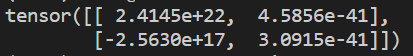torch.CPU Tensor类型(维度)：创建指定维度的Tensor，依旧有初始值，不过数据类型由方法名确定

# 创建维度(2,2)的Tensor，不过数据类型为IntTensor
y = torch.IntTensor(2, 2)
print(y.type())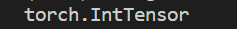2. torch.Tensor(list)：通过传入的listTensor内容初始化

import torch
l = list([[1, 2], [3, 4]])
# 利用l的内容进行初始化，若想换个数据类型则使用此类型对应的函数构造
x = torch.Tensor(l)
print(x)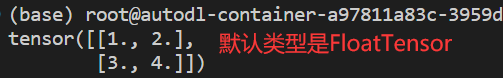## 2.1 特殊布局Tensor创建

import torch
l = list([[1, 1], [2, 2]])
x = torch.Tensor(l)
# 将x转换为torch.IntTensor类型
# 同样的，还有float()，double()，half()等方法可以进行对应转换
x = x.int()
print(x)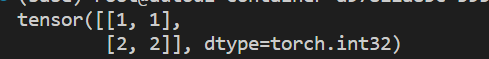1. torch.zeros(维度)：创建元素全为0Tensor

import torch
# 创建维度(2,2)的Tensor，不指定类型时默认都是FloatTensor，后续不再说明
x = torch.zeros(2,2)
print(x)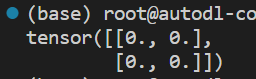2. torch.eye(维度)：创建对角线位置全1，其余位置全0Tensor

import torch
# 创建维度(3,3)的Tensor
x = torch.eye(3, 3)
print(x)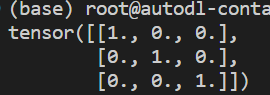3. torch.ones(维度)：创建元素全为1Tensor

import torch
x = torch.ones(3, 3)
print(x)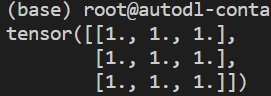4. torch.rand(维度)：创建将元素初始化为区间[0,1)的随机数Tensor

torch.randn(维度)：创建符合正态分布的随机数Tensor

import torch
x = torch.rand(3, 3)
print(x)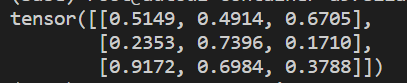5. torch.arange(start,end,step)：创建一个在区间[start,end)按指定步长step递增的一维Tensor

import torch
# 从1开始递增，步长为0.5，最后一个元素小于4
x = torch.arange(1, 4, 0.5)
print(x)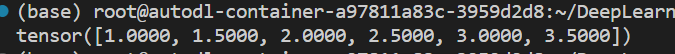6. torch.linspace(start,end,parts)：创建一个在区间[start,end]被均匀划分为parts份的一维Tensor

import torch
# 第一个元素是0，最后一个元素是10
# 总共要划分为5个元素，实际上间隔有4个，因此步长=(end-start)/4=2.5
x = torch.linspace(0, 10, 5)
print(x)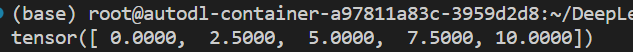7. torch.from_numpy(ndarray)：将Numpyndarray对象转换为Tensor

import torch
import numpy as np
arr = np.array([[1, 2], [3, 4]])
# 转换后的 Tensor 数据类型与 ndarry 一致
x = torch.from_numpy(arr)
print(x)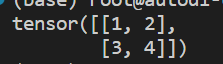# 3. Tensor数学操作

torch.add(a,n)张量a中每个元素加n，或与另一个张量n【维度相同】逐元素相加
torch.mul(a,n)张量a中每个元素乘n，或与另一个张量n【维度相同】逐元素相乘
torch.div(a,n)张量a中每个元素除n，或与另一个张量n【维度相同】逐元素相除
torch.fmod(a,n)torch.remainder(a,n)张量a中每个元素对n求余
torch.abs(a)张量a中的每个元素取绝对值
torch.ceil(a)张量a中的每个元素向上取整
torch.floor(a)张量a中的每个元素向下取整
torch.round(a)张量a中的每个元素取最近的整数【1.112.63
torch.frac(a)张量a中每个元素的分数部分
torch.neg(a)张量a中每个元素取负
torch.reciprocal(a)张量a中每个元素取倒数
torch.log(a)张量a中每个元素的自然对数【以e为底】
torch.pow(a,n)张量a中的每个元素取n次方
torch.exp(a)张量a中的每个元素变为指数，底数为e得到新值
torch.sigmoid(a)张量a中的每个元素代入sigmoid函数
torch.sign(a)张量a中若为正数或0，则1代替；否则-1代替
torch.sqrt(a)张量a中的每个元素取算数平方根，负数则放置nan
torch.dist(in,oth,p)张量in减去oth再求p范数【所有元素p次方之和再开p次方】
torch.mean(a)张量a中所有元素的均值
torch.norm(a)张量a的二范数【元素平方之和再开方】
torch.prod(a)张量a中所有元素之积
torch.sum(a)张量a中所有元素之和
torch.max(a)张量a中所有元素最大值
torch.min(a)张量a中所有元素最小值
torch.clamp(a,max,min)张量a中的元素大于max就取max，小于min就取min，其余不变
import torch
import numpy as np
l = list([[1, 2], [3, 4]])
x = torch.IntTensor(l)
# x中大于3的部分取3，小于2的部分取2
c = x.clamp(max=3, min=2)
print(c)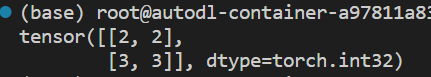# 4. Tensor线性代数

1. torch.dot(a,b)：向量a与向量b的点积【也叫内积】，结果是一个数
a ⃗ ⋅ b ⃗ = a 1 b 1 + a 2 b 2 + . . . + a n b n = ∑ i = 1 n a i b i \vec{a}\cdot\vec{b}=a_1b_1+a_2b_2+...+a_nb_n=\sum_{i=1}^{n}a_ib_i

import torch
a = torch.IntTensor([1, 2, 3])
b = torch.IntTensor([3, 2, 1])
# a向量与b向量点积
c = torch.dot(a, b)
print(c)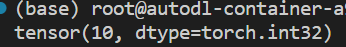2. torch.mv(a,b)：实现矩阵a与向量b的乘法【不需要手动转置操作】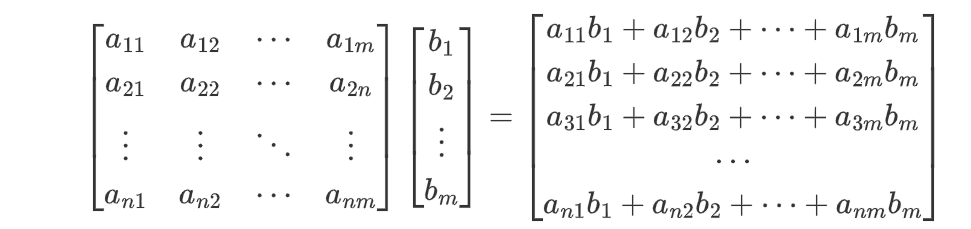import torch
a = torch.IntTensor([[1, 2, 3], [2, 3, 4], [3, 4, 5]])
b = torch.IntTensor([1, 2, 3])
# a矩阵与b向量相乘
c = torch.mv(a, b)
print(c)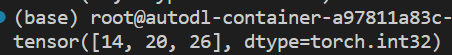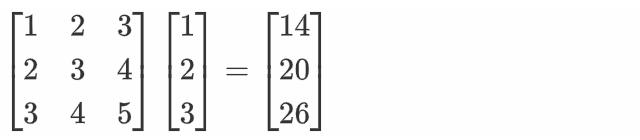3. torch.mm(a,b)：实现矩阵a与矩阵b相乘【矩阵乘法与向量类似，可以看作是多列向量】

import torch
a = torch.IntTensor([[1, 2, 3], [2, 3, 4], [3, 4, 5]])
b = torch.IntTensor([[2, 3, 4], [3, 4, 5], [4, 5, 6]])
# 矩阵a的第i行与矩阵b的第j列元素点积结果作为c矩阵第i行第j列的元素
c = torch.mm(a, b)
print(c)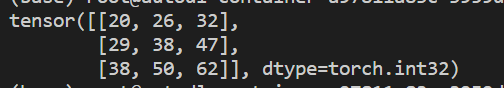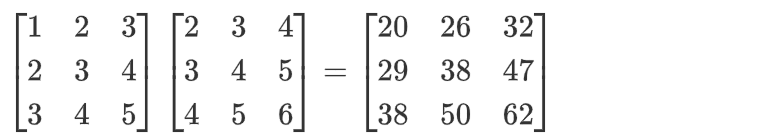torch.addmm(c,a,b)矩阵a与矩阵b相乘加上矩阵c
torch.addmv(b, a, c)矩阵a与向量b相乘加上向量c【向量是一维张量】
torch.addr(c,a,b)向量a与向量b求外积【列向量乘行向量】加上矩阵c
torch.bmm(b1,b2)两个batch内的矩阵进行批矩阵乘法【目前不知道啥意思】
torch.ger(a,b)求向量a与向量b的外积【即列向量与行向量相乘】
torch.inverse(a)求方阵a的逆矩阵
torch.addbmm(t,b1,b2)将两个batch内的矩阵b1,b2进行批矩阵乘法操作并累加，其结果与矩阵t相加
torch.baddbmm(t,b1,b2)将两个batch内的矩阵b1,b2进行批矩阵乘法操作，结果与另一batch内的矩阵t相加
torch.eig(a,eigenvectors=True)得到方阵a特征值以及对应的特征向量

import torch
a = torch.Tensor([[-1, 1, 1], [1, -1, 1], [1, 1, -1]])
# eigenvectors参数为True才能得到特征向量
# 求a矩阵的特征值与特征向量
b = torch.eig(a, eigenvectors=True)
print(b)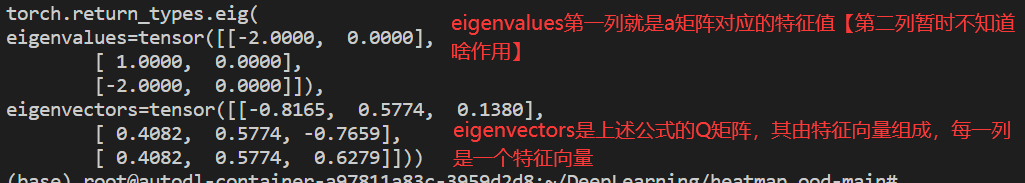import torch
# 原矩阵A
a = torch.Tensor([[-1, 1, 1], [1, -1, 1], [1, 1, -1]])
# 对矩阵A求特征值与特征向量
b = torch.eig(a, eigenvectors=True)
# 得到特征向量组成的矩阵Q
Q = b.eigenvectors
# 得到Q矩阵的逆矩阵
Q_inverse = torch.inverse(Q)
# 手动构建⋀矩阵。对角线是特征值，顺序为eigenvalues中特征值的顺序
e = torch.Tensor([[-2, 0, 0], [0, 1, 0], [0, 0, -2]])
# 计算Q矩阵乘⋀矩阵
f = torch.mm(Q, e)
# Q⋀矩阵再乘Q的逆矩阵
g = torch.mm(f, Q_inverse)
# 输出最终结果，若其与矩阵A一致，则说明我们上述判断正确
print(g)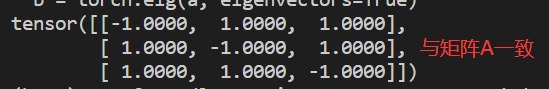# 5. Tensor连接与切片

• torch.cat((a,b,...),维度)：将张量a,b,...按照指定维度进行拼接【二维张量中，0代表行拼接，1是列拼接，换个说法就是第0维或者第1维】

可以这样理解，维度=0，即拼接后会让a[i]中的i变多【原来只有2行，拼接完变4行】；维度=1，即拼接后会让a[i][j]中的j变多【原来只有2列，拼接完变4列】。这种理解方式在高维张量中依然通用

import torch
a = torch.IntTensor([[1, 1], [1, 1]])
b = torch.IntTensor([[2, 2], [2, 2]])
# a与b张量按行拼接
c = torch.cat((a, b), 0)
print(c)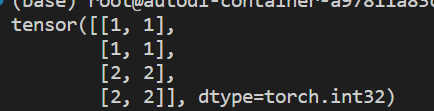• torch.chunk(a,parts,维度)：将张量a按照指定维度均分为parts块【如果不够分则会切分大小为1的块】，可以通过下标访问不同的块

# 将上述👆c行分割为2块
d = torch.chunk(c, 2, 0)
# 取第1块查看【其实就是张量a】
print(d)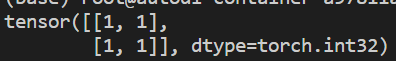• torch.t(a)：让矩阵a转置

import torch
a = torch.IntTensor([[1, 2], [3, 1]])
b = torch.t(a)
print(b)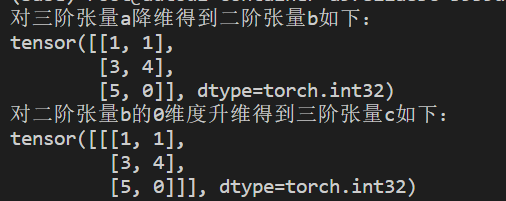• torch.split(a,parts,dim)：将张量a按照指定维度dim划分为parts块【此时与chunk相同】，parts也可以是tuple，此时每块大小由其内数字决定，没有分配到的会整体组成一大块

import torch
a = torch.IntTensor([[1, 1], [1, 1]])
b = torch.IntTensor([[2, 2], [2, 2]])
# a与b张量按行拼接
c = torch.cat((a, b), 0)
# 将c张量按行划分，第一块大小为3，剩下的所有行构成一块
d = torch.split(c, (3), 0)
print(d)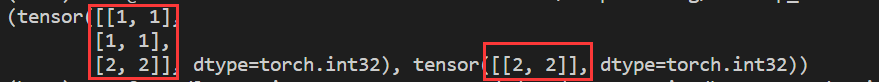• torch.index_select(a,dim,index)：张量adim维度方向按照index【类型是一阶Tensor】取对应元素

import torch
a = torch.IntTensor([[1, 2], [3, 4], [5, 6]])
b = torch.IntTensor([[2, 2], [2, 2]])
# 下标分别为0，2
index = torch.IntTensor([0, 2])
# 对张量a的第0维取index对应的元素，即a,a
c = torch.index_select(a, 0, index)
print(c)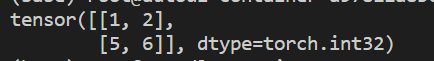• torch.unbind(a,dim)：张量a按照指定维度取切片，返回值是切片的Tensor集合【通俗来说，向量的切片是标量，矩阵切片是向量，三阶张量切片是矩阵（视觉上为“一根柱子”）】
假设有三阶张量 a m × n × v 则维度 = 0 的切片表达式为： ∑ j = 0 n ∑ k = 0 v a [ i ] [ j ] [ k ] 则维度 = 1 的切片表达式为： ∑ i = 0 m ∑ k = 0 v a [ i ] [ j ] [ k ] 则维度 = 2 的切片表达式为： ∑ i = 0 m ∑ j = 0 n a [ i ] [ j ] [ k ] 假设有三阶张量a_{m\times n\times v}\\ 则维度=0的切片表达式为：\sum_{j=0}^{n}\sum_{k=0}^{v}a[i][j][k]\\ 则维度=1的切片表达式为：\sum_{i=0}^{m}\sum_{k=0}^{v}a[i][j][k]\\ 则维度=2的切片表达式为：\sum_{i=0}^{m}\sum_{j=0}^{n}a[i][j][k]

import torch
a = torch.IntTensor([[1, 2], [3, 4], [5, 6]])
# 张量a按照维度=0进行切片
c = torch.unbind(a, 0)
print(c)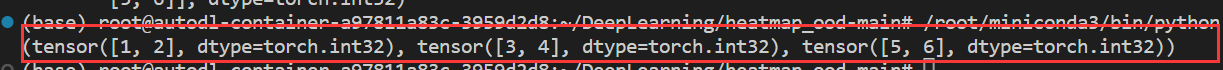• torch.nonzero(a)：返回张量a内值不为0的元素索引

import torch
a = torch.IntTensor([[1, 2], [3, 4], [5, 0]])
c = torch.nonzero(a)
print(c)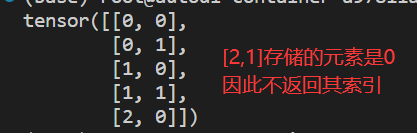• torch.squeeze(a)：对张量降维，如果当前维度是1，就将此维度处理掉

torch.unsqueeze(a,dim)：对张量升维，指定在dim维度升维

import torch
a = torch.IntTensor([[[1, 1], [3, 4], [5, 0]]])
b = torch.squeeze(a)
print('对三阶张量a降维得到二阶张量b如下：')
print(b)
c = torch.unsqueeze(b, 0)
print('对二阶张量b的0维度升维得到三阶张量c如下：')
print(c)• torch.transpose(a,dim1,dim2)：对a张量dim1维度与dim2维度进行转置
如张量 a m × n × v ， d i m 1 = 1 , d i m 2 = 2 则经过 t r a n s p o s e 操作后，张量 a 的结构变为 a m × v × m 如张量a_{m\times n\times v}，dim1=1,dim2=2\\ 则经过transpose操作后，张量a的结构变为a_{m\times v\times m}

import torch
a = torch.IntTensor([[[1, 1], [3, 4], [5, 0]]])
print('张量a的结构为：', a.shape)
# 对张量a的0维度与1维度转置
b = torch.transpose(a, 0, 1)
print('经转置后张量a的结构为：', b.shape)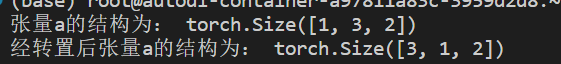# 6. Tensor变形

a.view(形状)：将张量a改变为指定形状，当使用-1作为某一维长度时，则这一维会被自动计算

import torch
# 张量a初始形状为[2,2,3]
a = torch.IntTensor(2, 2, 3)
# 将张量a的形状改变为[4,3]
b = a.view(4, 3)
print(b.shape)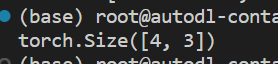# 7. Tensor自动微分

PytorchAutograd技术可以帮助我们自动求微分值

## 7.1 微分实例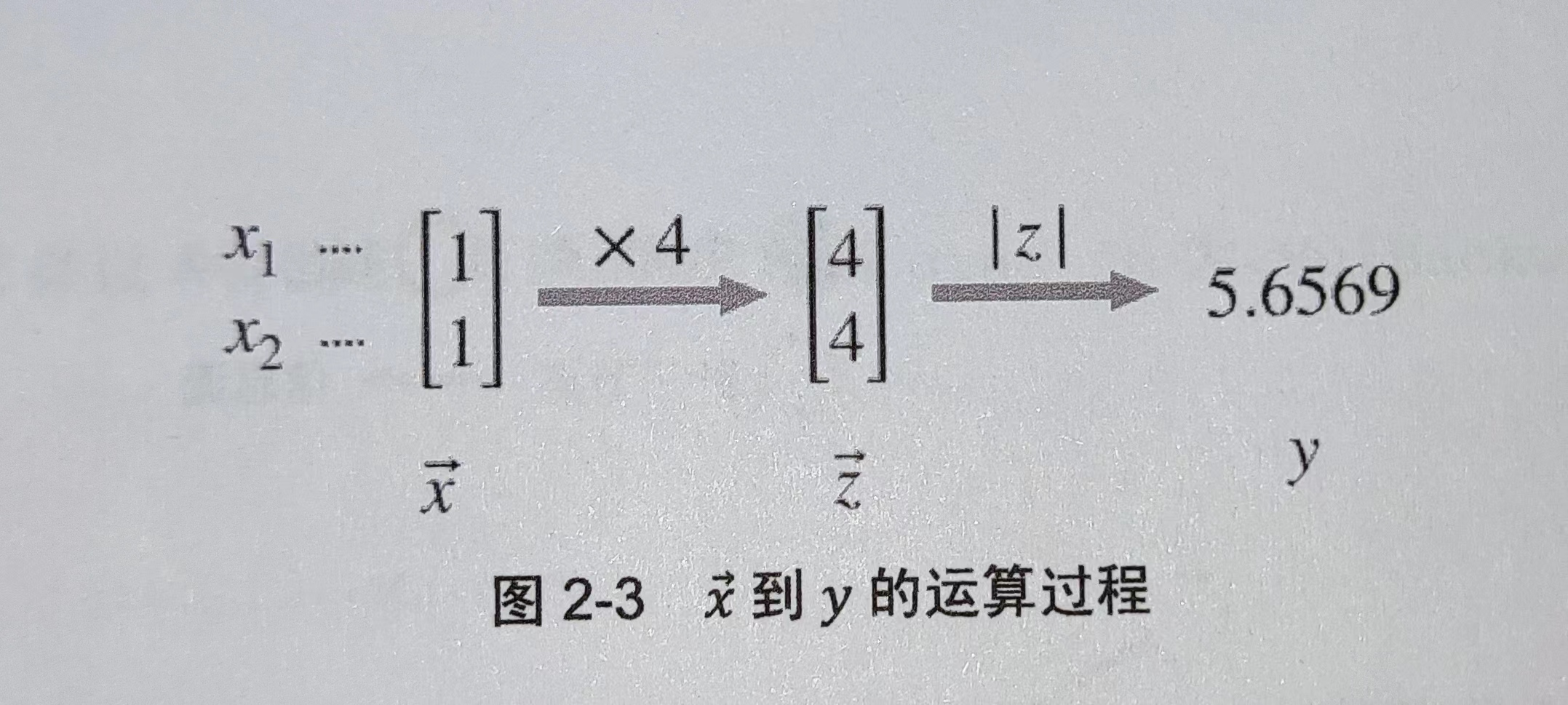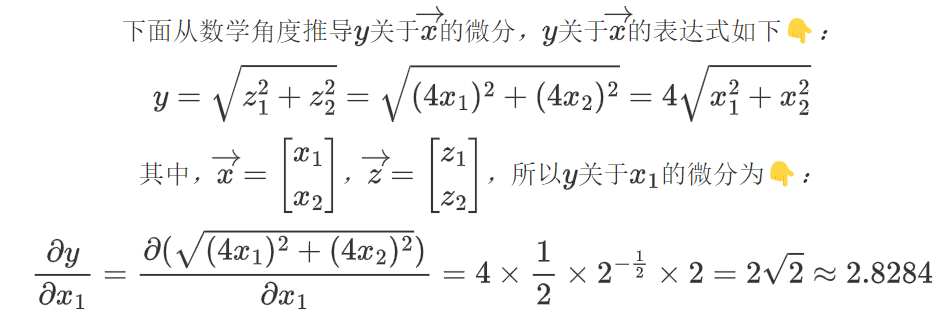## 7.2 基本原理

1. 叶子节点【图的末端，没有信息流经过，但信息流由此出发】
2. 中间节点【有信息流经过，信息流经过中间节点来到末端输出叶子节点】
3. 输出节点
4. 信息流【可以理解为有用信息集合，如上述求关于 x 1 x_1 的微分，此时 x 1 x_1 就说有用的信息】

Tensor在自动微分方面有三个重要属性👇：

• requires_grad：布尔值，默认为False，为True时表示此张量需要自动微分
• grad：存储张量微分值
• grad_fn：存储张量微分函数

## 7.3 代码示例

import torch
# x是一维张量且值全为 1
x = torch.ones(2)
# 我们后边需要计算 y 关于 x 的微分，因此 x 的 requires_grad 属性设置为 True
# 张量 x 的每个元素都乘 4 得到张量 z
z = x*4
# y 的值等于 z 的二阶范数
# 所谓二阶范数就是 张量内所有元素平方和再开方，与上述微分例子中一致
y = z.norm()
# y 启动反向传播，执行完毕后就能得到 y 关于张量 x 的微分【存储在 x 的 grad 中】
y.backward()### 462. 最小操作次数使数组元素相等 II——【Leetcode每日一题】

462. 最小操作次数使数组元素相等 II 给你一个长度为 n 的整数数组 nums &#xff0c;返回使所有数组元素相等需要的最小操作数。 在一次操作中&#xff0c;你可以使数组中的一个元素加 1 或者减 1 。 示例 1&#xff1a; 输入&#xff1a;nums [1,2,3] 输出&#xff1a;2 …

### PMP应该如何备考？

PMP现在是新考纲&#xff0c;PMP新版大纲加入了 ACP 敏捷管理的内容&#xff0c;而且还不少&#xff0c;敏捷混合题型占到了 50%&#xff0c;前不久官方也发了通知 8月启用第七版《PMBOK》&#xff0c;大家都觉得考试难度提升了&#xff0c;我从新考纲考完下来&#xff0c;最开…